A polygon with 4 sides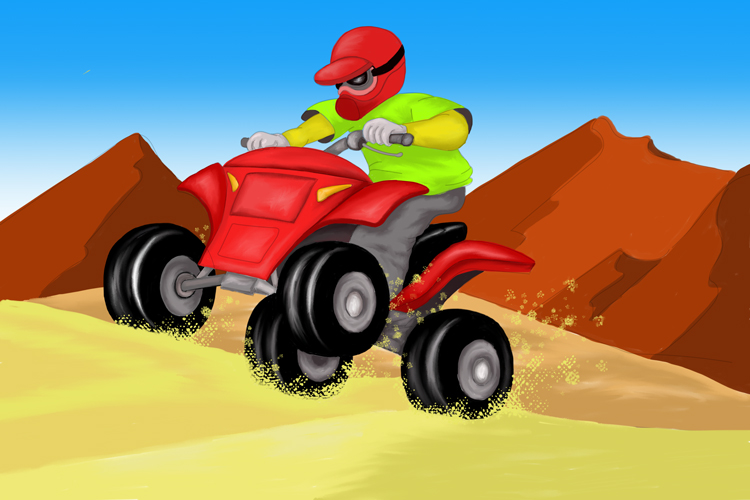Example 1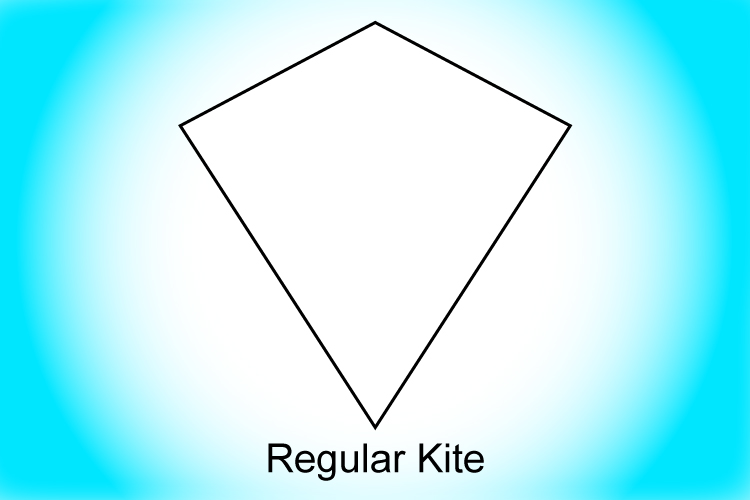A kite shape is an example of a quadrilateral.

Example 2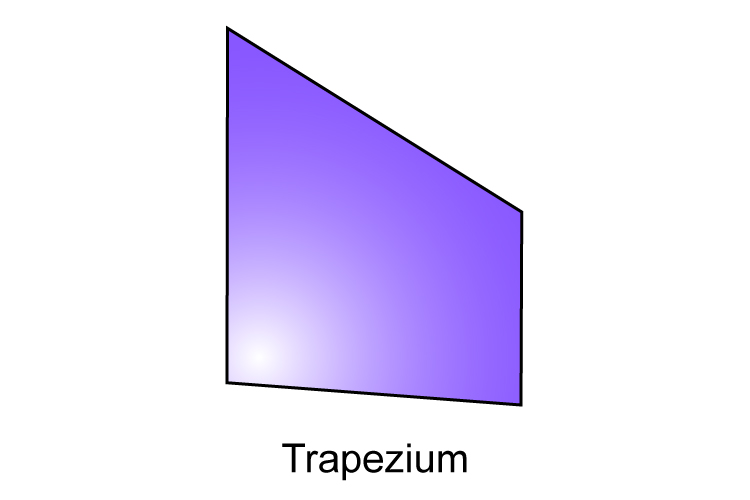A trapezium is a quadrilateral which has four sides where two of the opposing sides are parallel.

Example 3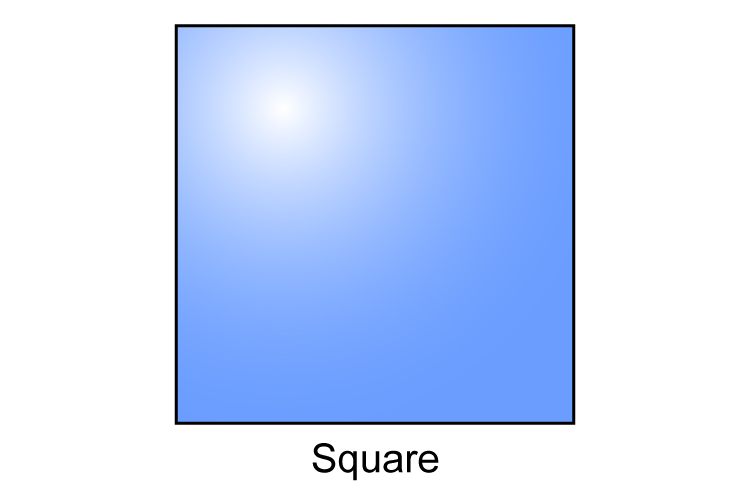A square is a quadrilateral which has four sides of equal length and an internal angle of 90° between each side.

Example 4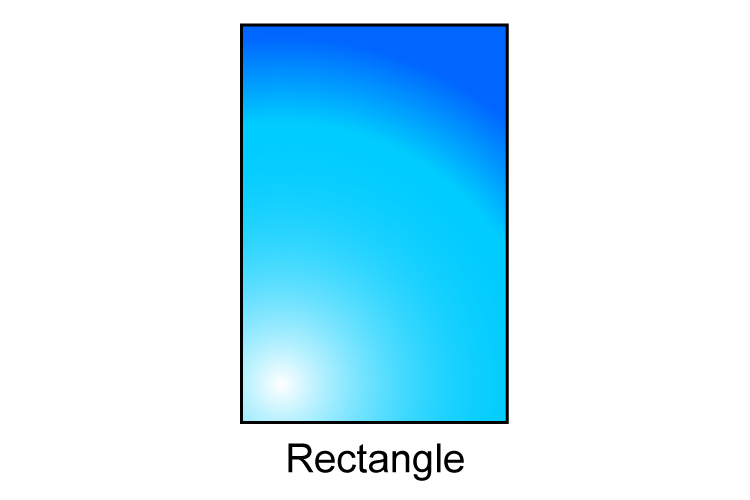A rectangle is a quadrilateral where each opposing side is parallel and of equal length, and the internal angle between each side is 90° but not all four sides are equal in length.

Example 5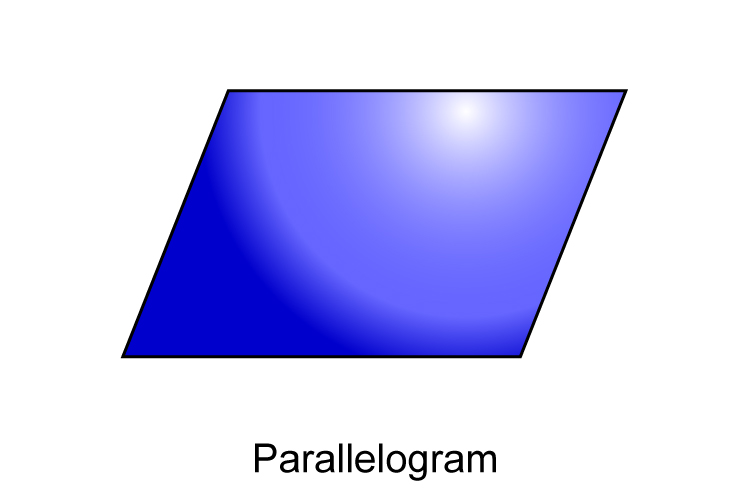A parallelogram is a quadrilateral where each opposing side is parallel and of equal length, but not all four sides are equal in length. None of the internal angles of a parallelogram are 90°.

Example 6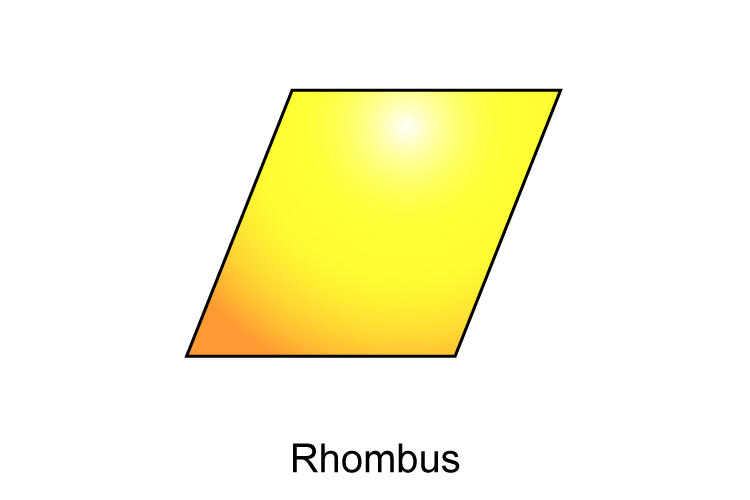A rhombus is a quadrilateral where each side is the same length and the opposing sides are parallel but the internal angle between each side is not 90°.